Finding point when tangent is parallel/ perpendicular

Chapter 6 Class 12 Application of Derivatives
Concept wise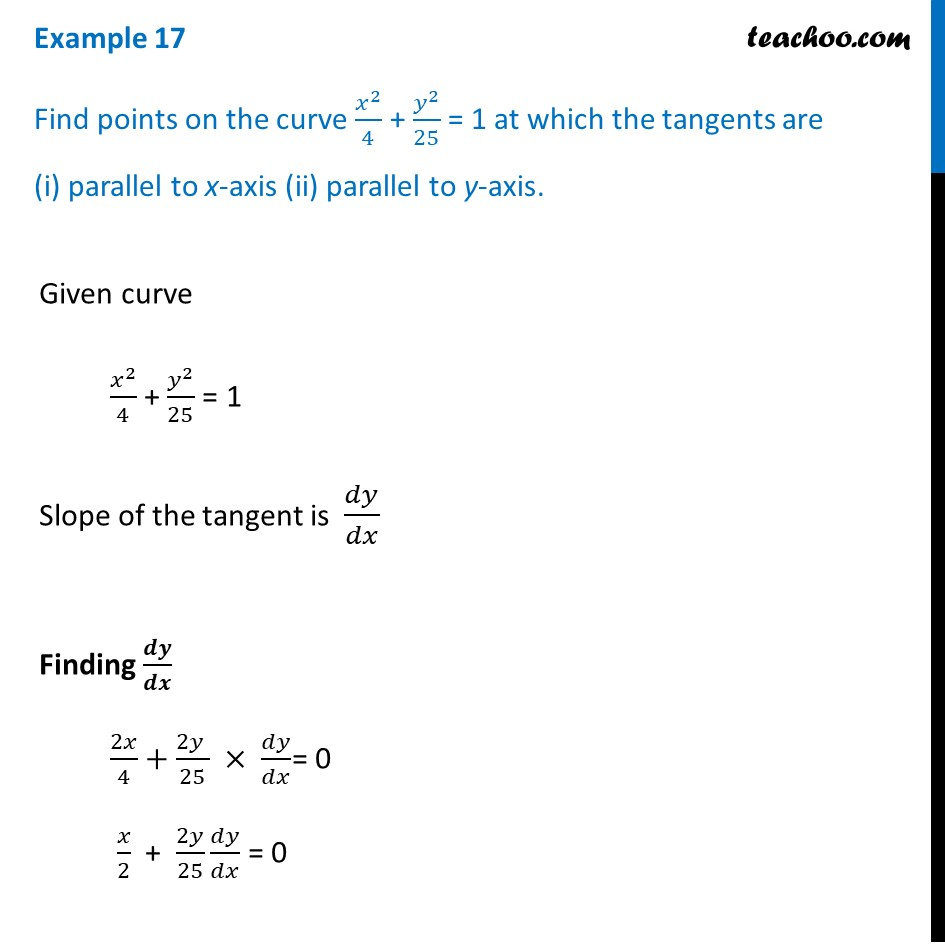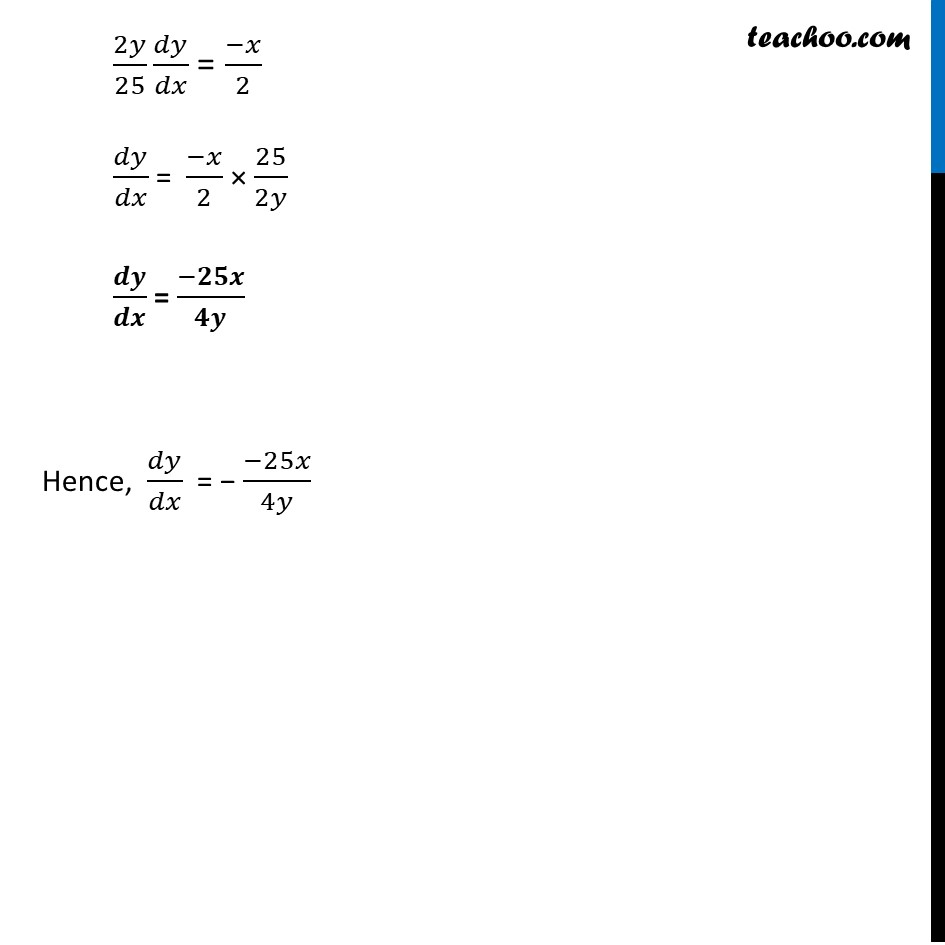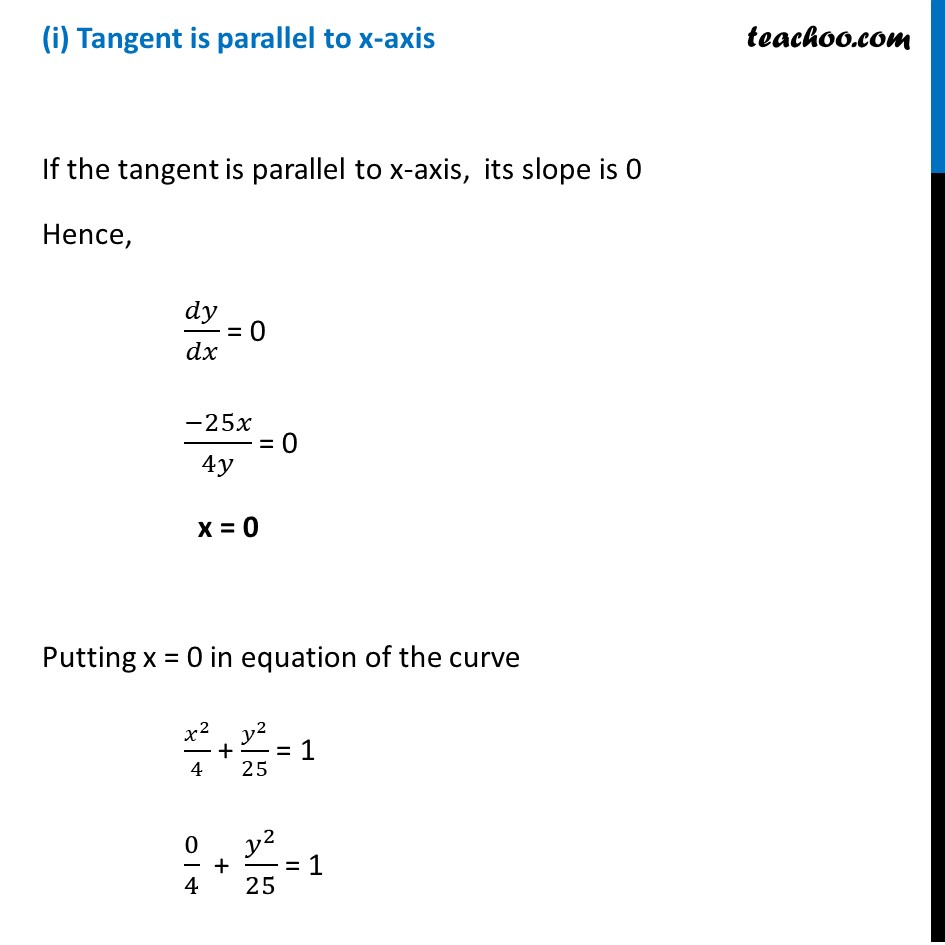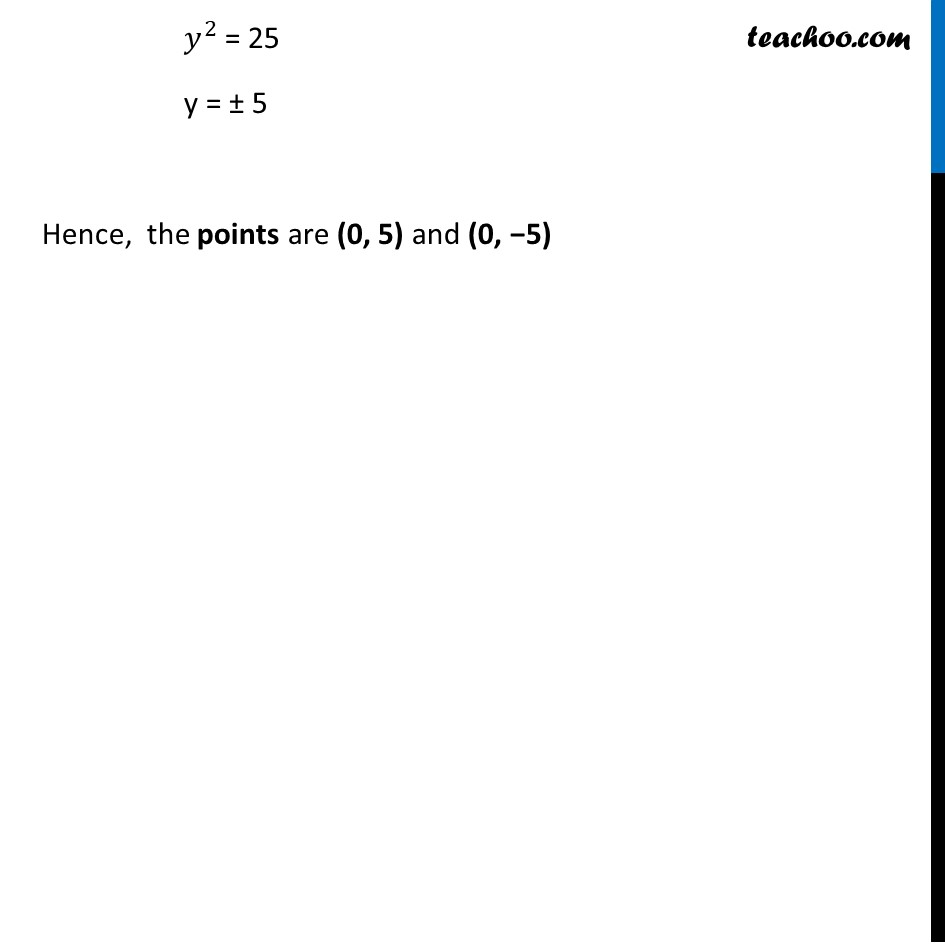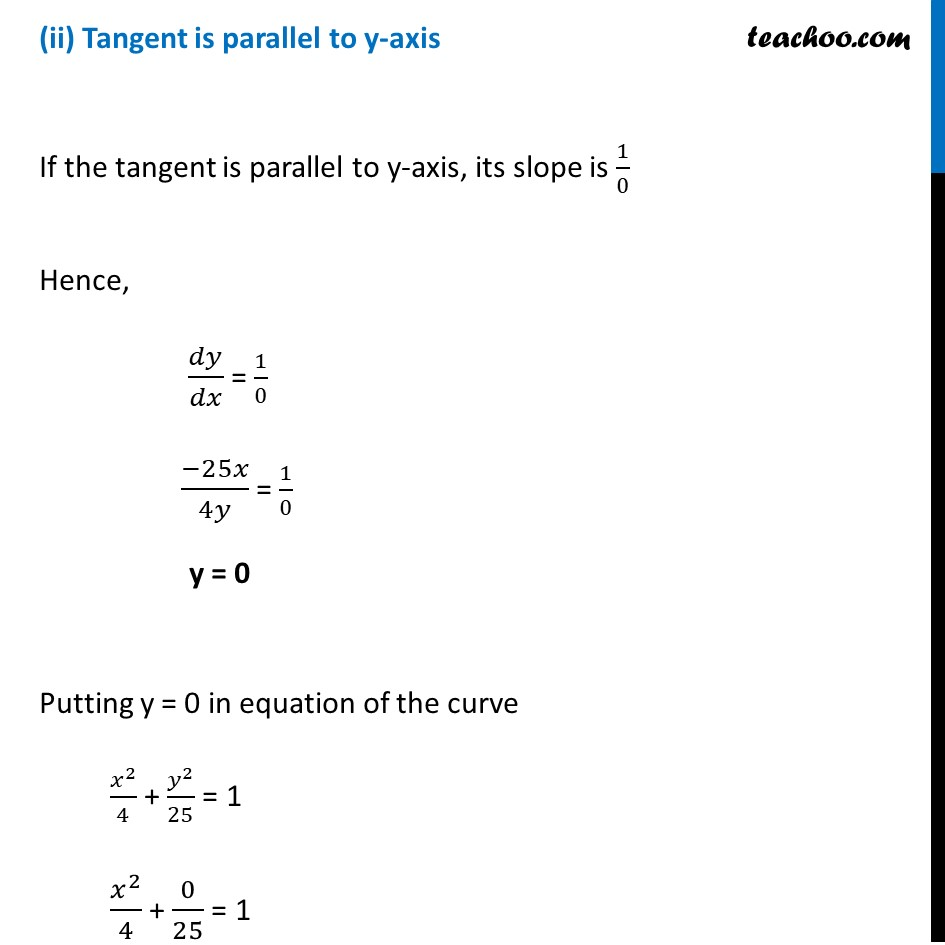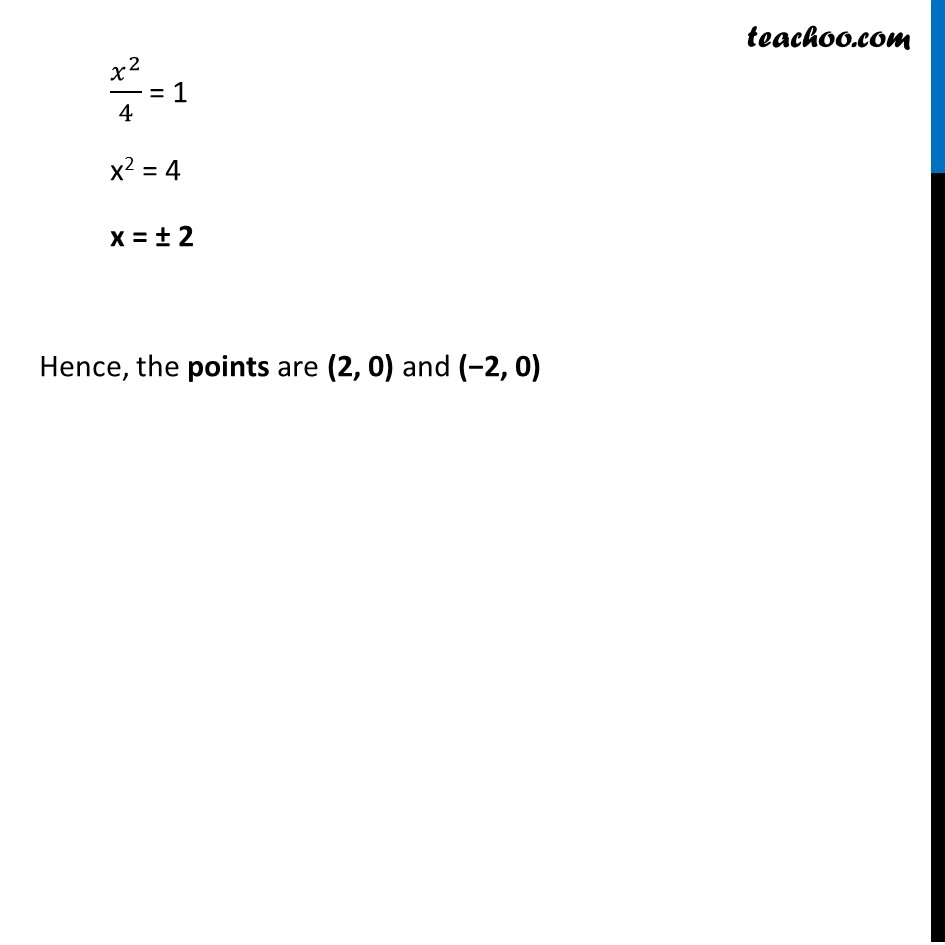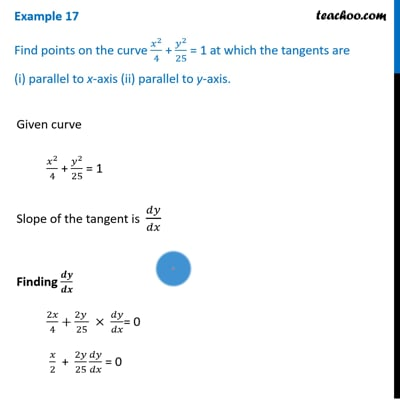This video is only available for Teachoo black users

Introducing your new favourite teacher - Teachoo Black, at only ₹83 per month

### Transcript

Example 17 Find points on the curve 𝑥^2/4 + 𝑦^2/25 = 1 at which the tangents are (i) parallel to x-axis (ii) parallel to y-axis.Given curve 𝑥^2/4 + 𝑦^2/25 = 1 Slope of the tangent is 𝑑𝑦/𝑑𝑥 Finding 𝒅𝒚/𝒅𝒙 2𝑥/4+(2𝑦 )/25 × 𝑑𝑦/𝑑𝑥= 0 𝑥/2 + 2𝑦/25 𝑑𝑦/𝑑𝑥 = 0 2𝑦/25 𝑑𝑦/𝑑𝑥 = (−𝑥)/2 𝑑𝑦/𝑑𝑥 = (−𝑥)/2 × 25/2𝑦 𝒅𝒚/𝒅𝒙 = (−𝟐𝟓𝒙)/𝟒𝒚 Hence, 𝑑𝑦/𝑑𝑥 = − (−25𝑥)/4𝑦 (i) Tangent is parallel to x-axis If the tangent is parallel to x-axis, its slope is 0 Hence, 𝑑𝑦/𝑑𝑥 = 0 (−25𝑥)/4𝑦 = 0 x = 0 Putting x = 0 in equation of the curve 𝑥^2/4 + 𝑦^2/25 = 1 0/4 + 𝑦^2/25 = 1 𝑦^2 = 25 y = ± 5 Hence, the points are (0, 5) and (0, −5) (ii) Tangent is parallel to y-axis If the tangent is parallel to y-axis, its slope is 1/0 Hence, 𝑑𝑦/𝑑𝑥 = 1/0 (−25𝑥)/4𝑦 = 1/0 y = 0 Putting y = 0 in equation of the curve 𝑥^2/4 + 𝑦^2/25 = 1 𝑥^2/4 + 0/25 = 1 𝑥^2/4 = 1 x2 = 4 x = ± 2 Hence, the points are (2, 0) and (−2, 0)